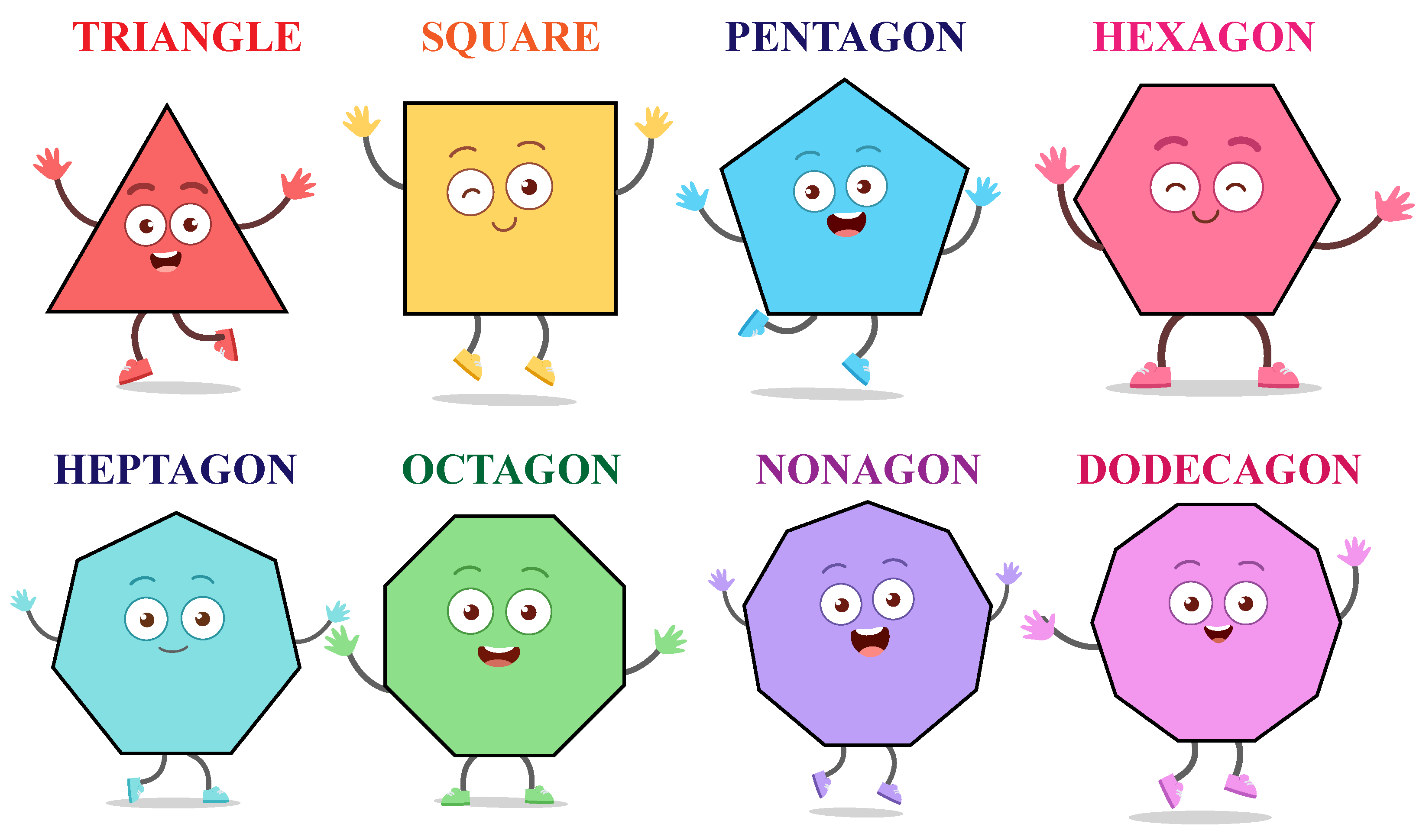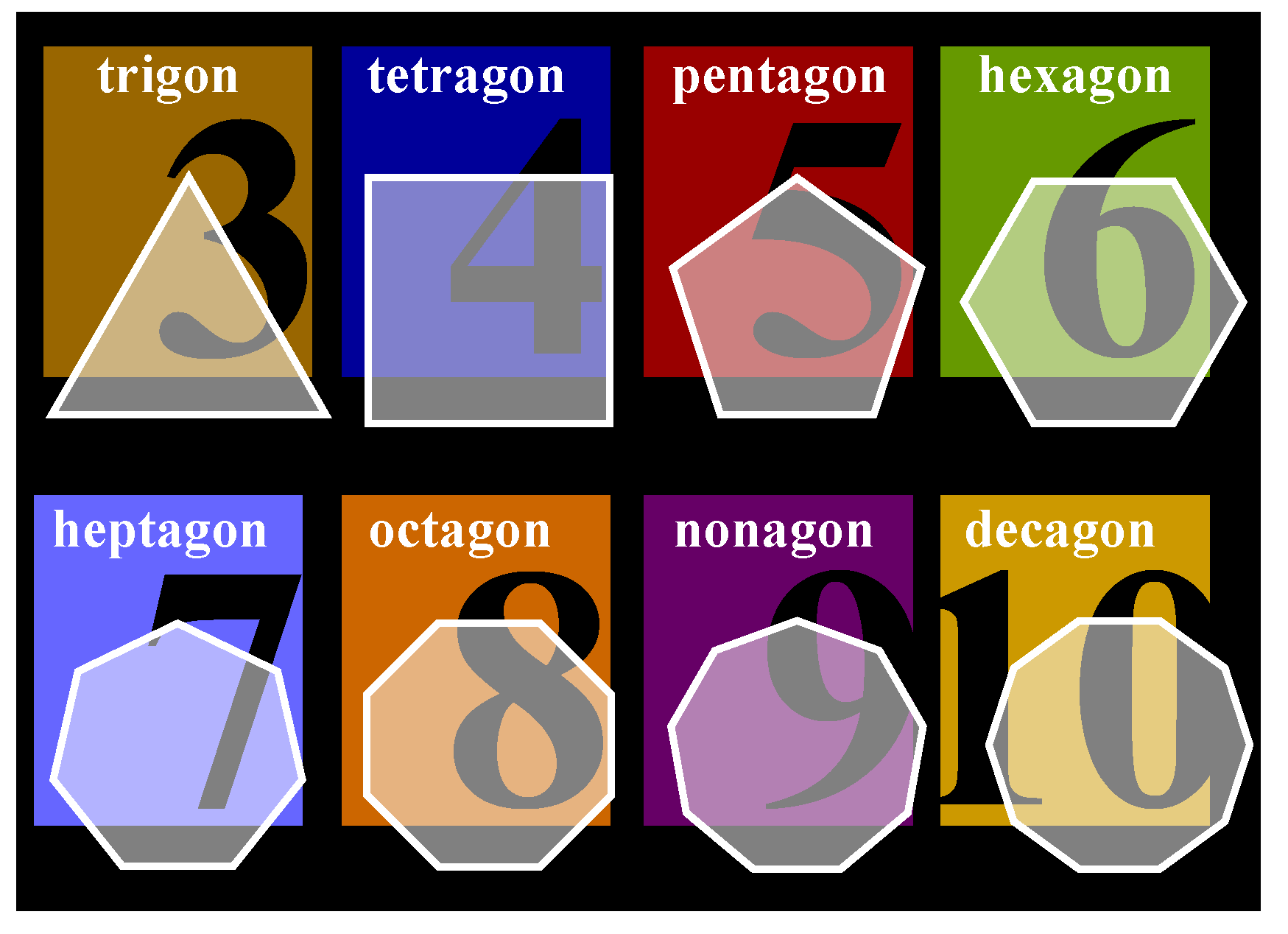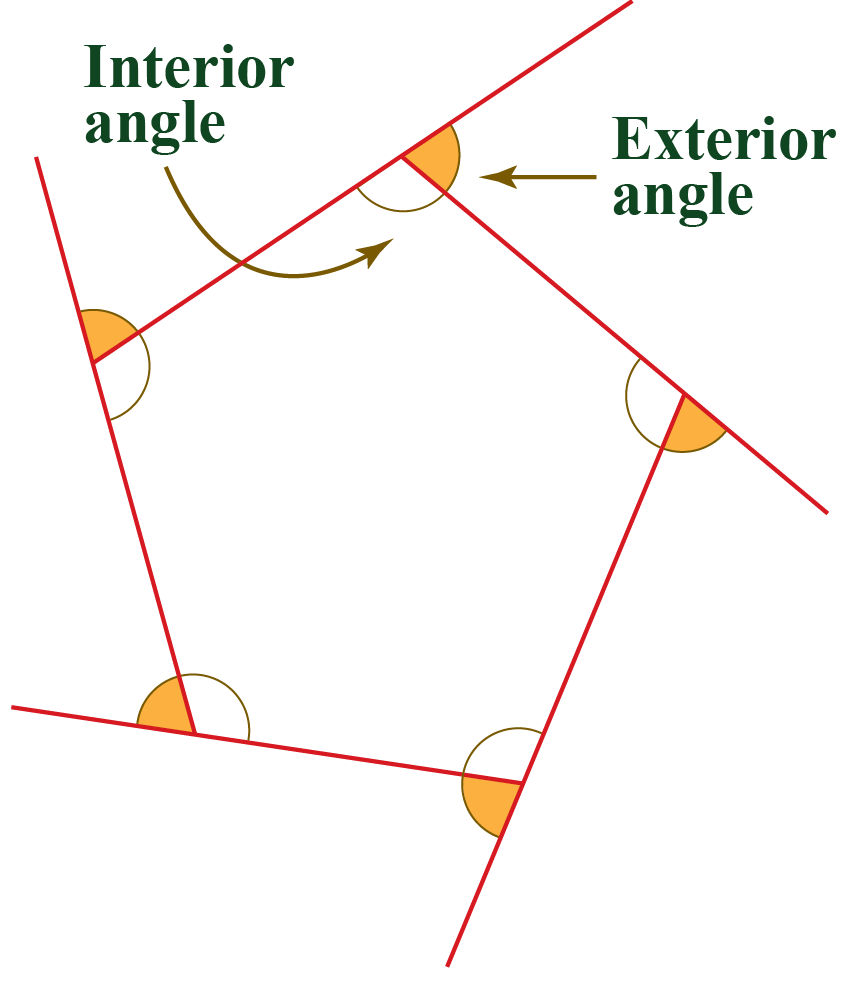# Polygon Shape

Sizes and shapes are the backbones of geometry. One of the most encountered shapes in geometry is polygons.

In this mini-lesson, we will explore the world of the polygon by finding the answers to the questions like what is a polygon, polygon shapes, polygon angles, sides of a polygon, and types of a polygon. Let us explore the chapter 'Polygon-Shapes' in detail.

## What Is A Polygon?

In geometry, a polygon is a closed two-dimensional figure with three or more straight lines.

The Greek word ‘Polygon’ consists of Poly meaning ‘many’ and gon meaning ‘angle.’

We see many different polygons around us.

For example, Have you ever seen a honeycomb? Can you guess ideally, how many sides a honeycomb could have?

A honeycomb is hexagonal in shape. You can very well relate the shape of a 6 sided polygon with a honeycomb.## Polygon Shape: Images

In geometry, we have different kinds of polygons with respect to the sides.

Have a look at the shapes below.These are some of the examples of polygon shapes

Each polygon is different in structure, they are categorized based on the number of sides and their properties.

### Polygon Chart

These are some of the familiar polygon shapes.## Polygon Angles

Look at the pentagon shape. It has two types of angles.• Interior angles - Angles measured between two sides of a polygon
• Exterior angles - Angles formed between any side to a line extended in its adjacent side.

The interior angles are formed inside and the exterior angles are formed outside the pentagon.

The following polygon formula can be used to find polygon angles.

 $$\text{Exterior Angle} = \frac{360^{\circ}}{n}$$
 $$\text{Interior angle} =\frac{180^{\circ}(n-2)}{n}$$

Where $$n$$ is number of sides.

Try your hands on this interesting simulation to know about angles of different shapes of a polygon.

More Important Topics
Numbers
Algebra
Geometry
Measurement
Money
Data
Trigonometry
Calculus